# NCERT solutions for Class 7 Maths chapter 12 - Algebraic Expressions [Latest edition]

#### Chapters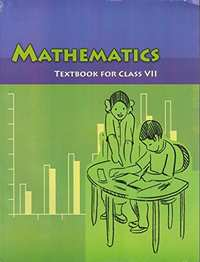## Chapter 12: Algebraic Expressions

Exercise 12.1Exercise 12.2Exercise 12.3Exercise 12.4
Exercise 12.1 [Pages 234 - 235]

### NCERT solutions for Class 7 Maths Chapter 12 Algebraic ExpressionsExercise 12.1 [Pages 234 - 235]

Exercise 12.1 | Q 1.1 | Page 234

Get the algebraic expressions in the following cases using variables, constants and arithmetic operation

Subtraction of z from y

Exercise 12.1 | Q 1.2 | Page 234

Get the algebraic expressions in the following cases using variables, constants and arithmetic operation

One-half of the sum of numbers x and y.

Exercise 12.1 | Q 1.3 | Page 234

Get the algebraic expressions in the following cases using variables, constants and arithmetic operation

The number z multiplied by itself.

Exercise 12.1 | Q 1.4 | Page 234

Get the algebraic expressions in the following cases using variables, constants and arithmetic operation

One-fourth of the product of numbers p and q.

Exercise 12.1 | Q 1.5 | Page 234

Get the algebraic expressions in the following cases using variables, constants and arithmetic operation

Numbers x and y both squared and added

Exercise 12.1 | Q 1.6 | Page 234

Get the algebraic expressions in the following cases using variables, constants and arithmetic operation

Number 5 added to three times the product of number m and n.

Exercise 12.1 | Q 1.7 | Page 234

Get the algebraic expressions in the following cases using variables, constants and arithmetic operation

Product of numbers y and z subtracted from 10.

Exercise 12.1 | Q 1.8 | Page 234

Get the algebraic expressions in the following cases using variables, constants and arithmetic operation

Sum of numbers and b subtracted from their product

Exercise 12.1 | Q 2.1 | Page 234

Identify the terms and their factors in the following expressions

Show the terms and factors by tree diagrams.

a) x − 3

b)1 + x + x2

c) y − y3

d) 5xy2 +   7x2y

e) − ab + 2b2 − 3a2

Exercise 12.1 | Q 2.2 | Page 234

Identify terms and factors in the expressions given below:

a) − 4x + 5

b) − 4x + 5y

c) 5y + 3y2

d) xy + 2x2y2

e) pq + q

f) 1.2 ab − 2.4 b + 3.6 a

g) 3/4 x + 1/4

h) 0.1p2 + 0.2q2

Exercise 12.1 | Q 3 | Page 235

Identify the numerical coefficients of terms (other than constants) in the following expressions:

1) 5 − 3t2

2) 1 + t + t2 + t3

3) x + 2xy+ 3y

4) 100m + 1000n

5) − p2q2 + 7pq

6) 1.2a + 0.8b

7) 3.14 r2

8) 2 (l + b)

9) 0.1y + 0.01 y2

Exercise 12.1 | Q 4.1 | Page 235

Identify terms which contain x and give the coefficient of x.

1) y2x + y

2) 13y2− 8yx

3) x + y + 2

4) 5 + z + zx

5) 1 + x+ xy

6) 12xy2 + 25

7) 7x + xy2

Exercise 12.1 | Q 4.2 | Page 235

Identify terms which contain y2 and give the coefficient of y2.

1) 8 - xy2

2) 5y2 + 7x

3) 2x2y - 15xy2 + 7y2

Exercise 12.1 | Q 5.01 | Page 235

Classify into monomials, binomials and trinomials

4y − 7z

Exercise 12.1 | Q 5.02 | Page 235

Classify into monomials, binomials and trinomials

y2

Exercise 12.1 | Q 5.03 | Page 235

Classify into monomials, binomials and trinomials.

x + y − xy

Exercise 12.1 | Q 5.04 | Page 235

Classify into monomials, binomials and trinomials

100

Exercise 12.1 | Q 5.05 | Page 235

Classify into monomials, binomials and trinomials

ab − a − b

Exercise 12.1 | Q 5.06 | Page 235

Classify into monomials, binomials and trinomials

5 − 3t

Exercise 12.1 | Q 5.07 | Page 235

Classify into monomials, binomials and trinomials

4p2q − 4pq2

Exercise 12.1 | Q 5.08 | Page 235

Classify into monomials, binomials and trinomials

7mn

Exercise 12.1 | Q 5.09 | Page 235

Classify into monomials, binomials and trinomials

z2 − 3z + 8

Exercise 12.1 | Q 5.1 | Page 235

Classify into monomials, binomials and trinomials

a2 + b2

Exercise 12.1 | Q 5.11 | Page 235

Classify into monomials, binomials and trinomials

z2 + z

Exercise 12.1 | Q 5.12 | Page 235

Classify into monomials, binomials and trinomials

1 + x + x2

Exercise 12.1 | Q 6.1 | Page 235

State whether a given pair of terms is of like or unlike term

1, 100

Exercise 12.1 | Q 6.2 | Page 235

State whether a given pair of terms is of like or unlike term

-7x, 5/2 x

Exercise 12.1 | Q 6.3 | Page 235

State whether a given pair of terms is of like or unlike terms.

− 29x, − 29y

Exercise 12.1 | Q 6.4 | Page 235

State whether a given pair of terms is of like or unlike terms.

14xy, 42yx

Exercise 12.1 | Q 6.5 | Page 235

State whether a given pair of terms is of like or unlike terms.

4m2p, 4mp2

Exercise 12.1 | Q 6.6 | Page 235

State whether a given pair of terms is of like or unlike terms.

12xz, 12 x2z2

Exercise 12.1 | Q 7.1 | Page 235

Identify like terms in the following:

−xy2, − 4yx2, 8x2, 2xy2, 7y, − 11x2, − 100x, −11yx, 20x2y, −6x2, y, 2xy,3x

Exercise 12.1 | Q 7.2 | Page 235

Identify like terms in the following:

10pq, 7p, 8q, − p2q2, − 7qp, − 100q, − 23, 12q2p2, − 5p2, 41, 2405p, 78qp, 13p2q, qp2, 701p2

Exercise 12.2 [Pages 239 - 240]

### NCERT solutions for Class 7 Maths Chapter 12 Algebraic ExpressionsExercise 12.2 [Pages 239 - 240]

Exercise 12.2 | Q 1.1 | Page 239

Simplify combining like terms: 21b − 32 + 7b − 20b

Exercise 12.2 | Q 1.2 | Page 239

Simplify combining like terms: - z2 + 13z2 − 5z + 7z3 − 15z

Exercise 12.2 | Q 1.3 | Page 239

Simplify combining like terms: p − (p − q) − q − (− p)

Exercise 12.2 | Q 1.4 | Page 239

Simplify combining like terms: 3a - 2b - ab - (a - b + ab) + 3ab + b - a

Exercise 12.2 | Q 1.5 | Page 239

Simplify combining like terms: 5x2y − 5x2 + 3y x2 − 3y2 + x2 − y2 + 8xy2 −3y2

Exercise 12.2 | Q 1.6 | Page 239

Simplify combining like terms: (3y2 + 5y - 4) - (8y - y2 - 4)

Exercise 12.2 | Q 2.01 | Page 239

Add: 3mn, − 5mn, 8mn, −4mn

Exercise 12.2 | Q 2.02 | Page 239

Add: t - 8tz, 3tz - z, z - t

Exercise 12.2 | Q 2.03 | Page 239

Add: -7mn + 5, 12mn + 2, 9mn - 8, -2mn - 3

Exercise 12.2 | Q 2.04 | Page 239

Add: a + b - 3, b - a + 3, a - b + 3

Exercise 12.2 | Q 2.05 | Page 239

Add: 14x + 10y - 12xy - 13, 18 - 7x - 10y + 8xy, 4xy

Exercise 12.2 | Q 2.06 | Page 239

Add: 5m - 7n, 3n - 4m + 2, 2m - 3mn - 5

Exercise 12.2 | Q 2.07 | Page 239

Add: 4x2y, - 3xy2, - 5xy2, 5x2y

Exercise 12.2 | Q 2.08 | Page 239

Add: 3p2q2 - 4pq + 5, - 10p2q2, 15 + 9pq + 7p2q2

Exercise 12.2 | Q 2.09 | Page 239

Add:ab - 4a, 4b - ab, 4a - 4b

Exercise 12.2 | Q 2.1 | Page 239

Add: x2 - y2 - 1 , y2 - 1 - x2, 1- x2 - y2

Exercise 12.2 | Q 3.1 | Page 240

Subtract: - 5y2 from y2

Exercise 12.2 | Q 3.2 | Page 240

Subtract: 6xy from − 12xy

Exercise 12.2 | Q 3.3 | Page 240

Subtract: (a - b) from (a + b)

Exercise 12.2 | Q 3.4 | Page 240

Subtract: a (b - 5) from b (5 - a)

Exercise 12.2 | Q 3.5 | Page 240

Subtract: -m2 + 5mn from 4m2 - 3mn + 8

Exercise 12.2 | Q 3.6 | Page 240

Subtract: -x2 + 10x - 5 from 5x - 10

Exercise 12.2 | Q 3.7 | Page 240

Subtract: 5a2 - 7ab + 5b2 from 3ab - 2a2 -2b2

Exercise 12.2 | Q 3.8 | Page 240

Subtract: 4pq - 5q2 - 3p2 from 5p2 + 3q2 - pq

Exercise 12.2 | Q 4.1 | Page 240

What should be added to x2 + xy + y2 to obtain 2x2 + 3xy?

Exercise 12.2 | Q 4.2 | Page 240

What should be subtracted from 2a + 8b + 10 to get - 3a + 7b + 16?

Exercise 12.2 | Q 5 | Page 240

What should be taken away from 3x2 - 4y2 + 5xy + 20 to obtain - x2 - y2 + 6xy + 20?

Exercise 12.2 | Q 6.1 | Page 240

From the sum of 3x - y + 11 and - y - 11, subtract 3x - y - 11.

Exercise 12.2 | Q 6.2 | Page 240

From the sum of 4 + 3x and 5 - 4x + 2x2, subtract the sum of 3x2 - 5x and -x2 + 2x + 5.

Exercise 12.3 [Page 242]

### NCERT solutions for Class 7 Maths Chapter 12 Algebraic ExpressionsExercise 12.3 [Page 242]

Exercise 12.3 | Q 1.1 | Page 242

If m = 2, find the value of m − 2

Exercise 12.3 | Q 1.2 | Page 242

If m = 2, find the value of 3m − 5

Exercise 12.3 | Q 1.3 | Page 242

If m = 2, find the value of 9 - 5m

Exercise 12.3 | Q 1.4 | Page 242

If m = 2, find the value of: 3m2 − 2m − 7

Exercise 12.3 | Q 1.5 | Page 242

If m = 2, find the value of: "5m"/2 - 4

Exercise 12.3 | Q 2.1 | Page 242

If p = −2, find the value of: 4p + 7

Exercise 12.3 | Q 2.2 | Page 242

If p = -2, find the value of −3p2 + 4p + 7

Exercise 12.3 | Q 2.3 | Page 242

If p = -2, find the value of -2p3 - 3p2 + 4p + 7

Exercise 12.3 | Q 3.1 | Page 242

Find the value of the following expressions, when x = − 1:

2x - 7

Exercise 12.3 | Q 3.2 | Page 242

Find the value of the following expressions, when x = - 1:

-x + 2

Exercise 12.3 | Q 3.3 | Page 242

Find the value of the following expressions, when x = -1:

x2 + 2x + 1

Exercise 12.3 | Q 3.4 | Page 242

Find the value of the following expressions, when x = − 1:

2x2 − x − 2

Exercise 12.3 | Q 4.1 | Page 242

If a = 2, b = − 2, find the value of a2 + b2

Exercise 12.3 | Q 4.2 | Page 242

If a = 2, b = − 2, find the value of a2 + ab + b2

Exercise 12.3 | Q 4.3 | Page 242

If a = 2, b = − 2, find the value of a2 − b2

Exercise 12.3 | Q 5.1 | Page 242

When a = 0, b = − 1, find the value of the given expressions 2a + 2b

Exercise 12.3 | Q 5.2 | Page 242

When a = 0, b = − 1, find the value of the given expressions 2a2 + b2 + 1

Exercise 12.3 | Q 5.3 | Page 242

When a = 0, b = − 1, find the value of the given expressions 2a2 b + 2ab2 + ab

Exercise 12.3 | Q 5.4 | Page 242

When a = 0, b = − 1, find the value of the given expressions a2 + ab + 2

Exercise 12.3 | Q 6.1 | Page 242

Simplify the expressions and find the value if x is equal to 2:

x + 7 + 4 (x - 5)

Exercise 12.3 | Q 6.2 | Page 242

Simplify the expressions and find the value if x is equal to 2 :

3 (x + 2) + 5x - 7

Exercise 12.3 | Q 6.3 | Page 242

Simplify the expressions and find the value if x is equal to 2:

6x + 5 (x − 2)

Exercise 12.3 | Q 6.4 | Page 242

Simplify the expressions and find the value if x is equal to 2:

4 (2x - 1) + 3+ 11

Exercise 12.3 | Q 7.1 | Page 242

Simplify these expressions and find their values if x = 3, a = − 1, b = − 2.

3x - 5 - x + 9

Exercise 12.3 | Q 7.2 | Page 242

Simplify these expressions and find their values if x = 3, a = − 1, b = − 2

2 - 8x + 4x + 4

Exercise 12.3 | Q 7.3 | Page 242

Simplify these expressions and find their values if x = 3, a = − 1, b = − 2

3a + 5 - 8a + 1

Exercise 12.3 | Q 7.4 | Page 242

Simplify these expressions and find their values if x = 3, a = − 1, b = − 2

10 - 3b - 4 - 5b

Exercise 12.3 | Q 7.5 | Page 242

Simplify these expressions and find their values if x = 3, a = − 1, b = − 2.

2a - 2b - 4 - 5 + a

Exercise 12.3 | Q 8.1 | Page 242

if z = 10, find the value of z3 − 3 (z − 10).

Exercise 12.3 | Q 8.2 | Page 242

If p = − 10, find the value of p2 − 2p − 100

Exercise 12.3 | Q 9 | Page 242

What should be the value of a if the value of 2x2 + x − a equals to 5, when x = 0?

Exercise 12.3 | Q 10 | Page 242

Simplify the expression and find its value when a = 5 and b = −3.

2 (a2 + ab) + 3 − ab

Exercise 12.4 [Pages 246 - 247]

### NCERT solutions for Class 7 Maths Chapter 12 Algebraic ExpressionsExercise 12.4 [Pages 246 - 247]

Exercise 12.4 | Q 1.1 | Page 246

Observe the patterns of digits made from line segments of equal length. You will find such segmented digits on the display of electronic watches or calculators.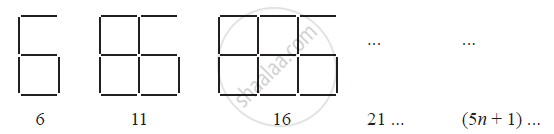If the number of digits formed is taken to be n, the number of segments required to
form n digits is given by the algebraic expression appearing on the right of each pattern.

How many segments are required to form 5, 10, 100 digits of the kind −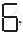Exercise 12.4 | Q 1.2 | Page 246

Observe the patterns of digits made from line segments of equal length. You will find such segmented digits on the display of electronic watches or calculators.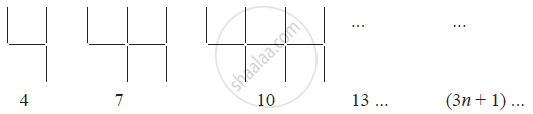If the number of digits formed is taken to be n, the number of segments required to form n digits is given by the algebraic expression appearing on the right of each pattern.

How many segments are required to form 5, 10, 100 digits of the kind −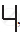Exercise 12.4 | Q 1.3 | Page 246

Observe the patterns of digits made from line segments of equal length. You will find such segmented digits on the display of electronic watches or calculators.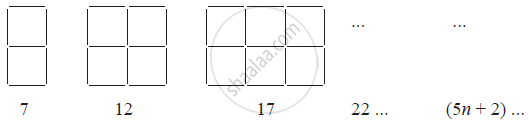If the number of digits formed is taken to be n, the number of segments required to form n digits is given by the algebraic expression appearing on the right of each pattern.

How many segments are required to form 5, 10, 100 digits of the kind −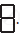Exercise 12.4 | Q 2 | Page 247

Use the given algebraic expression to complete the table of number patterns.

 S. No Expression Terms 1st 2nd 3rd 4th 5th ... 10th ... 100th ... 1 2n - 1 1 3 5 7 9 - 19 - - - 2 3n + 2 2 5 8 11 - - - - - - 3 4n + 1 5 9 13 17 - - - - - - 4 7n + 20 27 34 41 48 - - - - - - 5 n2 + 1 2 5 10 17 - - - - 10001 -

## Chapter 12: Algebraic Expressions

Exercise 12.1Exercise 12.2Exercise 12.3Exercise 12.4## NCERT solutions for Class 7 Maths chapter 12 - Algebraic Expressions

NCERT solutions for Class 7 Maths chapter 12 (Algebraic Expressions) include all questions with solution and detail explanation. This will clear students doubts about any question and improve application skills while preparing for board exams. The detailed, step-by-step solutions will help you understand the concepts better and clear your confusions, if any. Shaalaa.com has the CBSE Class 7 Maths solutions in a manner that help students grasp basic concepts better and faster.

Further, we at Shaalaa.com provide such solutions so that students can prepare for written exams. NCERT textbook solutions can be a core help for self-study and acts as a perfect self-help guidance for students.

Concepts covered in Class 7 Maths chapter 12 Algebraic Expressions are Algebraic Expressions, Terms, Factors and Coefficients of Expression, Like and Unlike Terms, Types of Algebraic Expressions as Monomials, Binomials, Trinomials, and Polynomials, Addition of Algebraic Expressions, Use of Variables in Common Rules, Evaluation of Algebraic Expressions by Substituting a Value for the Variable., Subtraction of Algebraic Expressions.

Using NCERT Class 7 solutions Algebraic Expressions exercise by students are an easy way to prepare for the exams, as they involve solutions arranged chapter-wise also page wise. The questions involved in NCERT Solutions are important questions that can be asked in the final exam. Maximum students of CBSE Class 7 prefer NCERT Textbook Solutions to score more in exam.

Get the free view of chapter 12 Algebraic Expressions Class 7 extra questions for Class 7 Maths and can use Shaalaa.com to keep it handy for your exam preparation